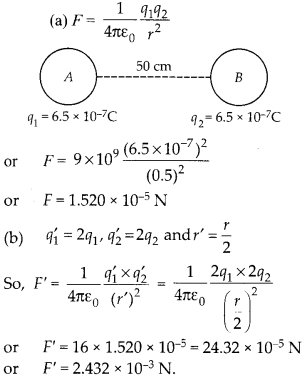Enlightened

# Question 12: NCERT Class 12th Physics: Chapter 1 – Electric Charges And Fields

• 0

Question 12: NCERT Class 12th Physics: Chapter 1 – Electric Charges And Fields

(a) Two insulated charged copper spheres A and B have their centres separated by a distance of 50 cm. What is the mutual force of electrostatic repulsion if the charge on each is 6.5 × 10-7 C ? The radii of A and B are negligible compared to the distance of separation.
(b) What is the force of repulsion if each sphere is charged double the above amount, and the distance between them is halved?

Share